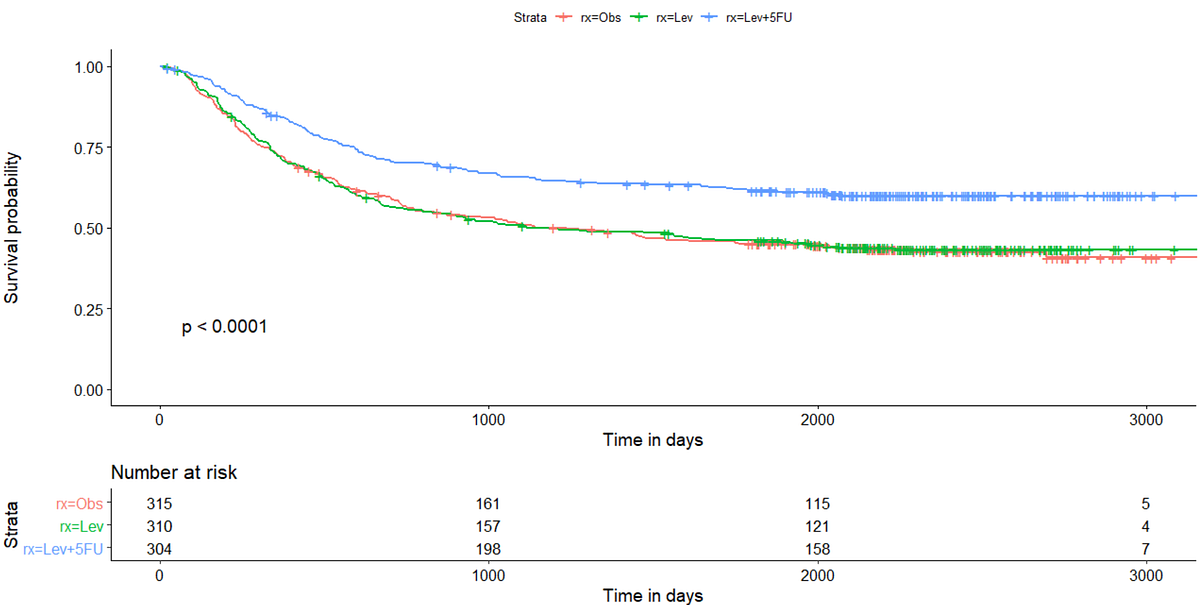# Interpreting Cox Proportional Hazards Model Using Colon Dataset in RInterpreting Cox Proportional Hazards Model Using Colon Dataset in R: Cox proportional hazards model is used to determine significant predictors for outcomes that are time-to-event.

Cox proportional hazards model is used to determine significant predictors for outcomes that are time-to-event. It is especially relevant in disciplines such as oncology, where outcomes are usually time-to-event (e.g overall survival and disease-free survival). Due to the complex nature of time-to-event outcome which involves censoring as well as both continuous and categorical components, it may be difficult to understand how to interpret the model initially. Hence, similar to what I did in my previous article on logistic regression, I would examine how to interpret R outputs for cox proportional hazards model as well as a test for proportional hazard assumption in the model.

Glossary for statistical terms for the later section:

1. Overall Survival: This is usually defined as from time to randomization to time to event, or last follow up if no event was observed throughout the period.
2. Hazard ratio: Similar to how odds is used in logistic regression, the equivalent for odds in cox proportional hazard model is hazard. The hazard ratio look into comparing the hazards occurring in one group in relation to the reference group (Eg. the experimental regimen vs standard treatment). Hazard ratio is the exponential form of the coefficients obtained in the Cox proportional hazard model.
3. Log rank test: This is to test for the overall difference in survival probability among the groups compared.

For the dataset, I will be using the colon dataset from the _survival _package. The data was collected from a clinical trial, which tested on the use of adjuvant chemotherapy regimens (Levamisole and Levamisole + 5-FU) for patients with colon cancer. While there are several variables in the dataset, we will be focusing on these variables to build the Cox proportional hazard model:

1. Treatment (rx): Whether patient was under Observation, Levamisole and Levamisole + 5-FU
2. Differentiation of the tumor (differ): Whether the tumor was considered as well differentiated, moderately differentiated or poorly differentiated
3. Nodal involvement (node4): Whether there were more than 4 nodes involved
4. Sex (sex): Whether patient was male or female
5. Time to event (time): Time taken for the event to occur, in this case death
6. Censoring status (censor): Whether the event was censored or not

## Data Manipulation In R | Data Manipulation In R With dplyr | R Programming For Beginners

This video on Data Manipulation in R will help you learn how to transform and summarize your data using different packages and functions. You will use the dplyr package to select, filter, arrange, and mutate data. You will use the tidyr library to create tidy data. You will look at functions such as gather, spread, separate, and unite. Let's begin!

## 7 steps to run a linear regression analysis using R

7 steps to run a linear regression analysis using R. I learned how to do regression analysis in R using brute force. With these 7 copy and paste steps, you can too.

## Data Types In R

Data types are kept easy. Data types of R are quite different when we compare with other programming languages. Here, we’ll outline the data types of R.

## R For Data Science Full Course | Data Science With R Full Course |Data Science Tutorial

Learn the essential concepts in data science and understand the important packages in R for data science. You will look at some of the widely used data science algorithms such as Linear regression, logistic regression, decision trees, random forest, including time-series analysis. Finally, you will get an idea about the Salary structure, Skills, Jobs, and resume of a data scientist.

## Unpivot a column of delimited data with R

Afer explaining how to unpivot columns of delimeted data in Power Query and Python, today I’m extending those explanations to R.